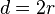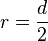# Diameter facts for kids

Kids Encyclopedia Facts

In geometry, the diameter of a circle is a line from one side directly to the opposite side through the centre. It can also be defined as the longest chord of a circle. The same explanations can be also used to describe the diameter in a sphere.

The letter d or the symbol are often used as a symbol for the diameter. The diameter's length is often called the diameter itself.

The diameter's length is twice the radius, and therefore the relationship between the diameter's length and radius can be shown with the equations:$d = 2r$ and$r = \frac{d}{2}$

## Related pagesDiameter Facts for Kids. Kiddle Encyclopedia.